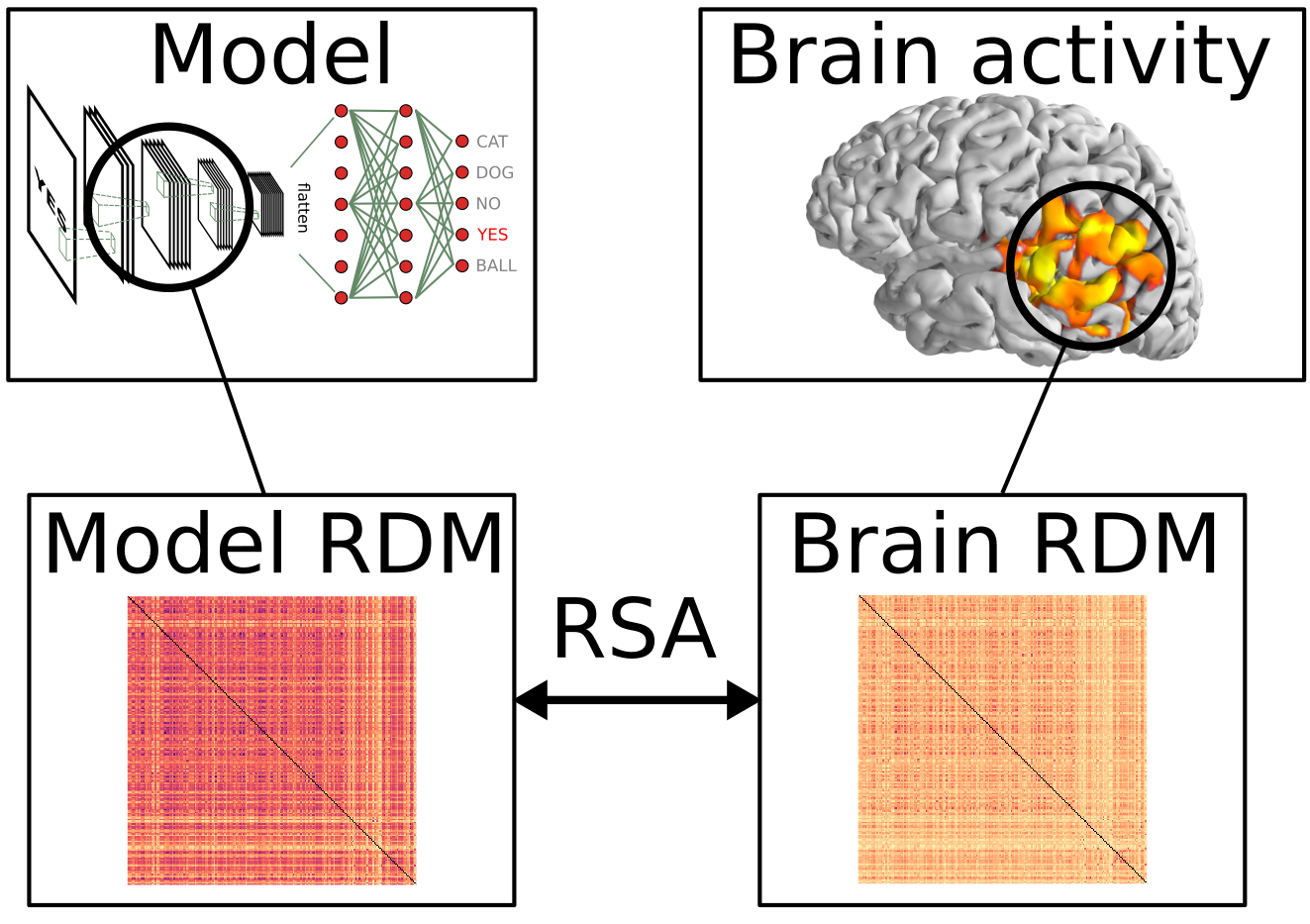# Representational Similarity Analysis¶

This is a Python package for performing representational similarity analysis (RSA) using MNE-Python data structures. The RSA is computed using a “searchlight” approach.

Read more on RSA in the paper that introduced the technique:

Nikolaus Kriegeskorte, Marieke Mur and Peter Bandettini (2008). Representational similarity analysis - connecting the branches of systems neuroscience. Frontiers in Systems Neuroscience, 2(4). https://doi.org/10.3389/neuro.06.004.2008# Installation¶

The package can be installed either through PIP: `pip install mne-rsa` or through conda using the conda-forge channel: `conda install -c conda-forge mne-rsa`

# Use cases¶

This is what the package can do for you:

• Compute DSMs on arbitrary data

• Compute DSMs in a searchlight across:

• vertices/voxels and samples (source level)

• sensors and samples (sensor level)

• vertices/voxels only (source level)

• sensors only (sensor level)

• samples only (source and sensor level)

• Use cross-validated distance metrics when computing DSMs

• And of course: compute RSA between DSMs

Supported metrics for comparing DSMs:

• Spearman correlation (the default)

• Pearson correlation

• Kendall’s Tau-A

• Linear regression (when comparing multiple DSMs at once)

• Partial correlation (when comparing multiple DSMs at once)

# Juicy bits of the API¶

```def compute_dsm(data, metric='correlation', **kwargs)

stc_dsm_metric='correlation', stc_dsm_params=dict(),
rsa_metric='spearman', y=None, n_folds=1, sel_vertices=None,
tmin=None, tmax=None, n_jobs=1, verbose=False):

evoked_dsm_params=dict(), rsa_metric='spearman', y=None,
n_folds=1, picks=None, tmin=None, tmax=None, n_jobs=1,
verbose=False):

epochs_dsm_params=dict(), rsa_metric='spearman', y=None,
n_folds=1, picks=None, tmin=None, tmax=None, n_jobs=1,
verbose=False):

image_dsm_metric='correlation', image_dsm_params=dict(),
```

# Example usage¶

Basic example on the EEG “kiloword” data:

```import mne
import rsa
data_path = mne.datasets.kiloword.data_path(verbose=True)
# Compute the model DSM using all word properties
evoked_rsa = rsa.rsa_epochs(epochs, dsm_model,
verbose=True)
```

# Documentation¶

For quick guides on how to do specific things, see the examples.

Finally, there is the API reference documentation.

# Integration with other packages¶

I mainly wrote this package to perform RSA analysis on MEG data. Hence, integration functions with MNE-Python are provided. There is also some integration with nipy for fMRI.

# Performance¶

This package aims to be fast and memory efficient. An important design feature is that under the hood, everything operates on generators. The searchlight routines produce a generator of DSMs which are consumed by a generator of RSA values. Parallel processing is also supported, so you can use all of your CPU cores.

# Development¶

Here is how to set up the package as a developer:

```git clone git@github.com:wmvanvliet/mne-rsa.git
cd mne-rsa
python setup.py develop --user
```